Author Message
cnodnarb
Valued Contributor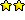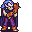Joined: 11 Sep 2002
Posts: 688
Location: Evansville, INPosted: Fri May 06, 2011 7:51 pm    Post subject: Window Spy/IE again.... SOLVED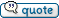EDIT See next post.
This was more difficult than I thought it would be.

Sometimes I feel like a genius, sometimes I feel like a dunce.

Getting the Internet Explorer internal window is still tripping me up.

Can someone elegantinize this code and/or test your browsers to make sure it works and report back?

Code updated to get rid of repeat/until and some count fixes.

NOTE: Nothing wrong with repeat/until, unless your a fan of goto. Which I am.

Still very very ugly code. Seems to work though, tested 9 and 6 so far. Getting ready to test 7. Anyone have 8?

EDIT - Failed Internet Explorer 7 *sigh*.

The problem is it doesn't enumerate all the children at each level. Has anyone solved this problem before? I think I've seen it...

 Code: :loop      %1 = @window(@winexists(#ieframe),child) if @greater(%%next,-1) %%count = -1 :loop1 if @equal(%%count,%%next) goto next1 end %1 = @window(%1,next) %%count = @succ(%%count) goto loop1 :next1    end      :loop2      if @null(%1)      goto incrementnext      end      if %1          %x = @winclass(%1)  if @equal(@winclass(%1),Internet Explorer_server)      goto found      end      %1 = @window(%1,child)      if %1          %x = @winclass(%1)      if @equal(@winclass(%1),Internet Explorer_server)      goto found      end      end      end      if @null(%1)      if %2      %1 = @window(%2,next)      if %1          %x = @winclass(%1)      if @equal(@winclass(%1),Internet Explorer_server)      goto found      end      end      end      end      %2 = %1      if %1      %x = @winclass(%1)  if @equal(@winclass(%1),Internet Explorer_server)      goto found      end      end      goto loop2     :incrementnext     if @null(%%next)     %%next = 0     else %%next = @succ(%%next) end goto loop :found info %1

Last edited by cnodnarb on Fri May 06, 2011 10:05 pm; edited 2 times in total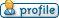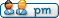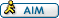cnodnarb
Valued ContributorJoined: 11 Sep 2002
Posts: 688
Location: Evansville, INPosted: Fri May 06, 2011 9:54 pm    Post subject:Updated to handle tabbed windows. Returns all bound window separated by @cr()
 Code: %a = @window(@winexists(#ieframe),child) %b = @succ(%b) %c = 2 list create,%b list add,%b,%a repeat     if %a         %a = @window(%a,next)         list add,%b,%a     end until @null(%a) repeat     list seek,%b,0     list create,%c     %%list = %b     %%index = 0     %%count = @count(%%list)     repeat         list seek,%%list,%%index             if @item(%b)                 %a = @window(@winexists(@item(%b)),child)                     if %a                         list add,%c,%a                         repeat                             if %a                                 %a = @window(%a,next)                                 list add,%c,%a                             end                         until @null(%a)                     end             end         %%index = @succ(%%index)     until @equal(%%index,%%count)     %b = @succ(%b)     %c = @succ(%c) until @null(@text(%b)) %b = @succ(%b) list create,%b %%match = "Internet Explorer_Server" %%list = 0 repeat %%list = @succ(%%list) %%index = 0 %%count = @count(%%list)     repeat     list seek,%%list,%%index         if @item(%%list)             if @equal(@winclass(@item(%%list)),%%match)                     list add,%b,@item(%%list)                     end                                end             end         end         %%index = @succ(%%index)     until @equal(%%index,%%count) until @equal(%%list,@diff(%b,2)) info @text(%b)cnodnarb
Valued ContributorJoined: 11 Sep 2002
Posts: 688
Location: Evansville, INPosted: Mon May 06, 2013 7:11 pm    Post subject:Code: %%win2 = #shell_traywnd %%win3 = rebarwindow32 %a = @window(@winexists(%%win2),child) %b = 1 %c = 2 if @equal(%%firstpass,true) list create,%b else list clear,%b end list add,%b,%a repeat     if %a         %a = @window(%a,next)         list add,%b,%a     end until @null(%a) repeat     list seek,%b,0     if @equal(%%firstpass,true) list create,%c else list clear,%c end     %%list = %b     %%index = 0     %%count = @count(%%list)     repeat         list seek,%%list,%%index             if @item(%b)                 %a = @window(@winexists(@item(%b)),child)                     if %a                         list add,%c,%a                         repeat                             if %a                                 %a = @window(%a,next)                                 list add,%c,%a                             end                         until @null(%a)                     end             end         %%index = @succ(%%index)     until @equal(%%index,%%count)     %b = @succ(%b)     %c = @succ(%c) until @null(@text(%b)) %b = @succ(%b) if @equal(%%firstpass,true) list create,%b else list clear,%b end %%match = %%win3 %%list = 0 repeat %%list = @succ(%%list) %%index = 0 %%count = @count(%%list)     repeat     list seek,%%list,%%index         if @item(%%list)             if @equal(@winclass(@item(%%list)),%%match)                     list add,%b,@item(%%list)                     end                                end             end         end         %%index = @succ(%%index)     until @equal(%%index,%%count) until @equal(%%list,@diff(%b,2)) rem info @text(%b) %%rebar = @trim(@text(%b)) %%firstpass = falseDisplay posts from previous: All Posts1 Day7 Days2 Weeks1 Month3 Months6 Months1 Year Oldest FirstNewest First
 All times are GMT Page 1 of 1